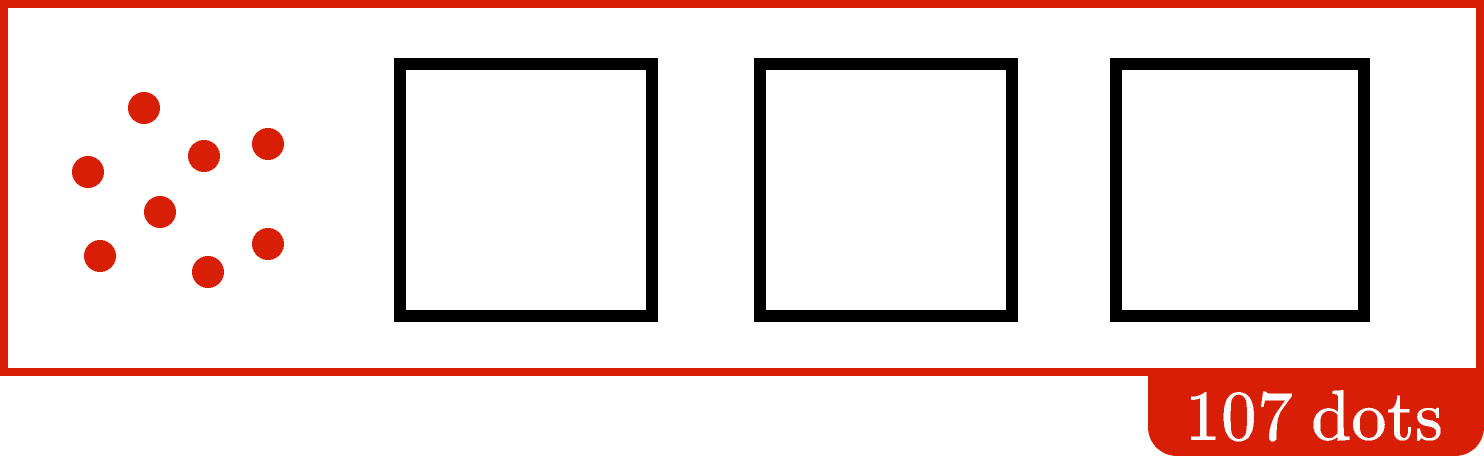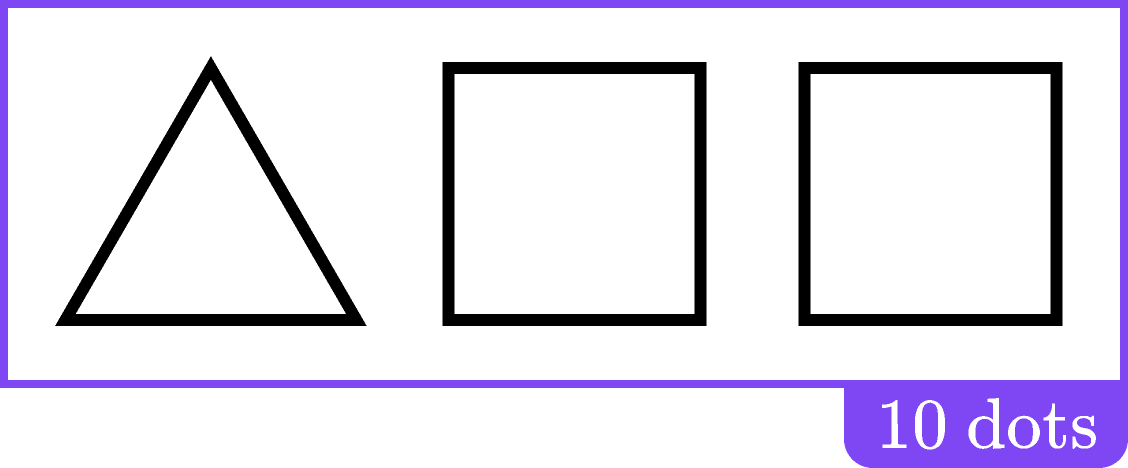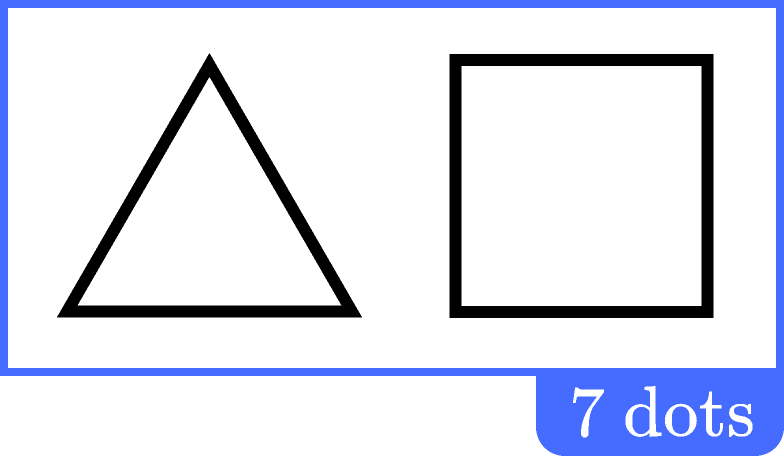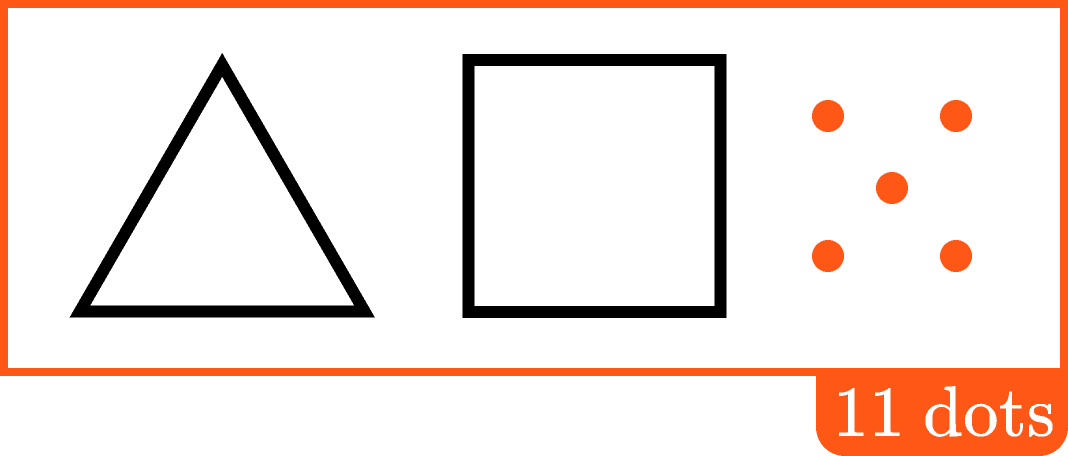### Pre-Algebra

We ended the last quiz with a dot puzzle, where the number of dots per square was a variable that we could adjust to change the total number of dots in the image.

In this quiz we continue working with dot puzzles, to develop our intuition for solving equations with variables. We will even go so far as to solve problems with more than one variable, without needing to use any formal algebraic methods!

First, we'll warm up with another problem where you can find the solution by experimenting with a slider.

# Dot Puzzles

Three squares each contain an identical number of dots.

How many dots should go in each square so that the total number of dots $($including the $4$ outside of the squares$)$ is $13?$

# Dot Puzzles

In the previous problem, the number of dots per square was a variable serving as an unknown quantity. If we represent the number of dots per square with the letter $D,$ then the problem was asking when

$4 + D + D + D = 13$

or, equivalently,

$4 + 3 \times D = 13$

is true.

To solve, we were able to simplify the problem into an arithmetic calculation: first, subtracting $4$ from $13,$ and then dividing the result by $3.$

The dot puzzles that follow don't include a slider; try to work out how you might solve the problem with arithmetic, rather than experimenting with dots.

# Dot Puzzles

Three squares each contain an identical number of dots.How many dots should go in each square so that the total number of dots (including those outside of the squares) is $107?$

# Dot Puzzles

Both squares contain the same number of dots. The number of dots in a square and the number of dots in a triangle may or may not be the same.

Two squares and one triangle together have $10$ dots. How many dots must be in the triangle?# Dot PuzzlesUsing $T$ and $S$ to stand for the number of dots in triangles and squares, respectively, the last problem could be represented by

$T + S + S = 10$

As there are multiple values of $T$ that work, there is no set "solution." This does not mean the algebra is meaningless! A variable is not just a box waiting for a number; it can be used to indicate relationships between numbers, without having to fix what those numbers are. If I state

"My brother is five years older than me."

both "my brother's age" and "my age" are variables with a set relationship, and this might be the only information given.

This can have practical value; for instance, in a scientific study involving siblings who are five years apart in age, the study might want to make a statement about this relationship that applies no matter what the specific ages are.

# Dot Puzzles

If given a relationship without a clear solution as in the previous question, adding more information may be enough to find one.

Two squares and one triangle together have $10$ dots:Additionally, just one square and one triangle together have $7$ dots:With all rules as before, where each square contains the same number of dots and each triangle contains the same number of dots, how many dots are in each square?

# Dot Puzzles

The rules here are the same as before (all squares have the same number of dots, as do all triangles).

Including the dots that are not in a triangle or square, there are $11$ dots in the following figure:Two squares and one triangle together have $10$ dots:How many dots are in each square?

# Dot Puzzles

We have now seen how to solve dot puzzles without using the slider to guess and check our answers, even after complicating the puzzles with an additional variable. And while we connected some puzzles with the practice of using letters to represent variables, we didn't need to use those letters to solve the problems, demonstrating that algebra is not simply arithmetic with letters.

As problems get more abstract, we will see that representing variables with letters can streamline the problem-solving process. However, this requires us to first be comfortable with the meanings behind some of the formal rules of algebra. In the following chapters, we will discover those meanings using what we already know about geometry and arithmetic!

# Dot Puzzles

×

Problem Loading...

Note Loading...

Set Loading...